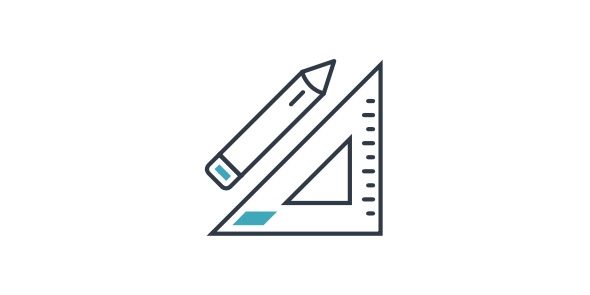# Erhs Grade 6 Math Quiz

5 Questions | Total Attempts: 400SettingsThis is a grade 6 math quiz. Its a grade 6 math quiz

• 1.
If you have 17 green squares, 11 purple sguares and 14 blue squares, what is the ratio of blue squares to purple squares.
• A.

17:11

• B.

11:17

• C.

14:17

• D.

17:14

• E.

14:11

• 2.
What does14 830+239 786 equal?
• A.

244 617

• B.

244 616

• C.

244 716

• D.

244 716

• E.

244 615

• 3.
Which ones are equivelant fractions?
• A.

17/3 and 5 2/3

• B.

17/2 and 5 1/3

• C.

11/4 and 5 1/3

• D.

18/3 and 6 1/4

• 4.
Whats the right answer?2x3(4+6)3x6-4=(hint: use BDMAS)
• A.

30

• B.

29

• C.

31

• D.

33

• E.

34

• 5.
What is 34x28?
• A.

950

• B.

951

• C.

952

• D.

953

• E.

954

Related TopicsBack to top